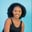Related Tags

python
numpy

# How to use np.subtract() for a 2D array in PythonMaria Elijah

## Overview

The Python library Numpy provides us with a method called subtract(). We use this to subtract two arrays.

### The numpy.subtract() method

​​The numpy.subtract() method returns the element-wise (element by element) difference between two arrays.

We can create a two-dimensional (2D) array using a list of lists in Python.

### Syntax

numpy.subtract(A, B, dtype=None, out=None)


### Parameters

• A: This is the first input array.
• B: This is the second input array.
• dtype: This is an optional parameter. It represents the return type of the array.
• out: This is an optional parameter. It represents the alternative output array where the result is to be placed.

Note: If A and B have distinct shapes, they must be able to be broadcasted to a common shape for output representation.

### Return value

The numpy.subtract() method returns the difference between two input arrays, element-wise.

If the out parameter is specified, this method returns an array reference to out.

### Code

The following code shows how to use np.subtract() for 2D arrays.

# Import numpy.
import numpy as np
# Create two 2D arrays.
A = np.array([[2,6,5],[3,4,8]])
B = np.array([[1,7,2],[10,9,4]])

# Find the difference between the 2D arrays.
# Store the result in arr_diff.
arr_diff = np.subtract(A, B)

print(arr_diff)
Using np.subtract() for 2D arrays in Python

### Explanation

• Line 2: We import the numpy library.
• Line 4–5: We create two 2D arrays, A and B.
• Line 9: We use the np.subtract() method to find the difference between the x1 and x2 arrays. The result is stored in a new variable called arr_diff.
• Line 11: We display the result to the console.

RELATED TAGS

python
numpy

CONTRIBUTORMaria Elijah
RELATED COURSES

View all Courses

Keep Exploring

Learn in-demand tech skills in half the time